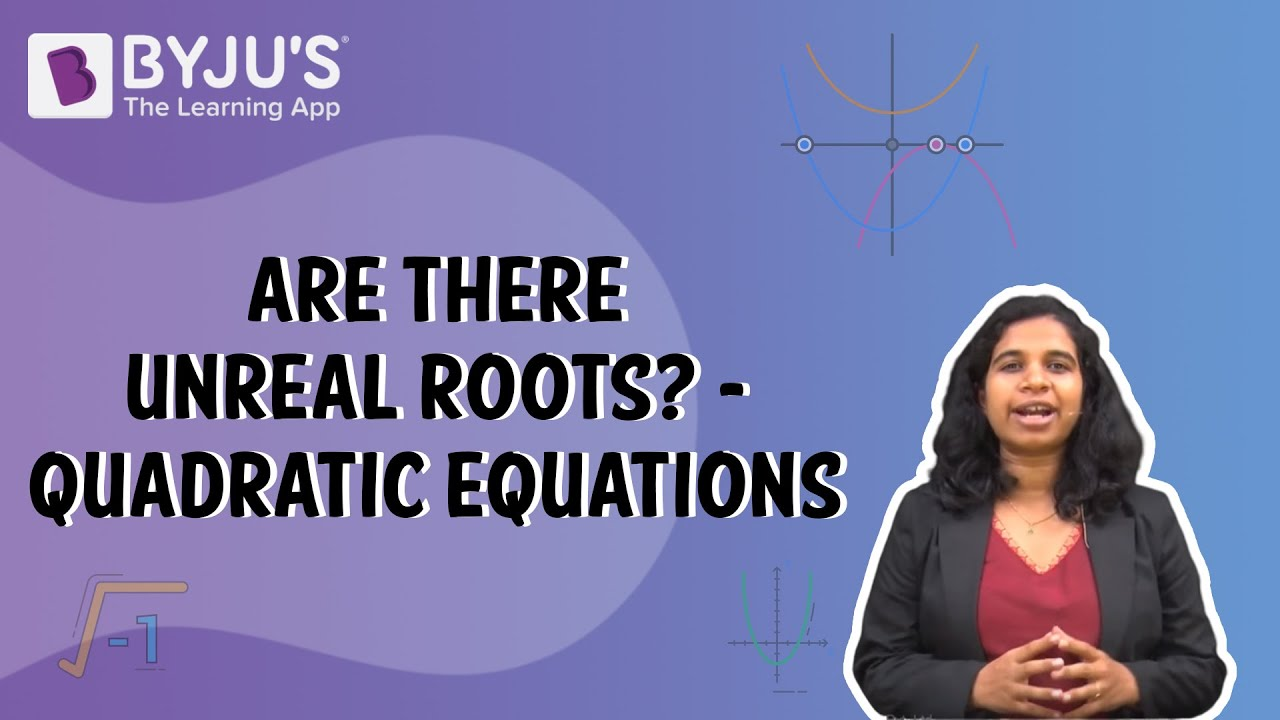# Are there unreal roots in quadratic equations?

Quadratic equations can be defined as a polynomial equation of a second degree, which implies that it comprises a minimum of one term that is squared. The general form of the quadratic equation is:

ax² + bx + c = 0

where

x is an unknown variable and a,b,c are numerical coefficients.

The values of variables satisfying the given quadratic equation are called their roots. In other words, x = α is a root of the quadratic equation f(x), if f(α) = 0.

The real roots of an equation f(x) = 0 are the x-coordinates of the points where the curve y = f(x) intersect the x-axis.

• One of the roots of the quadratic equation is zero and the other is -b/a if c = 0
• Both the roots are zero if b = c = 0
• The roots are reciprocal to each other if a = c

### Discriminant

The term (b2 – 4ac) in the quadratic formula is known as the discriminant of a quadratic equation. The discriminant of a quadratic equation reveals the nature of roots.

### Nature of roots of quadratic equation

 Value of Discriminant Nature of roots 1 If the value of discriminant = 0 i.e. b2 – 4ac = 0 The quadratic equation will have equal roots i.e. α = β = -b/2a 2 If the value of discriminant < 0 i.e. b2 – 4ac < 0 The quadratic equation will have imaginary roots i.e α = (p + iq) and β = (p – iq). Where ‘iq’ is the imaginary part of a complex number 3 If the value of discriminant (D) > 0 i.e. b2 – 4ac > 0 The quadratic equation will have real roots 4 If the value of discriminant > 0 and D is a perfect square The quadratic equation will have rational roots 5 If the value of discriminant (D) > 0 and D is not a perfect square The quadratic equation will have irrational roots i.e. α = (p + √q) and β=(p – √q) 6 If the value of discriminant > 0, D is a perfect square, a = 1 and b and c are integers The quadratic equation will have integral roots.

Factorization is possible for any quadratic equations with real roots. The roots have physical significance because the graph of an equation contacts the x-axis at the roots. In the Cartesian plane, the x-axis symbolises the real line. This means that if an equation has unreal roots, it will not intersect the x-axis and hence cannot be factored.